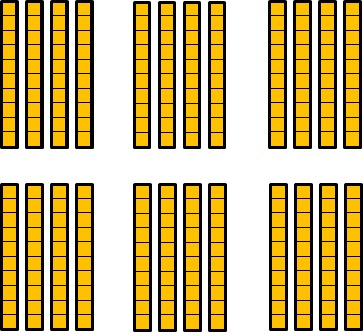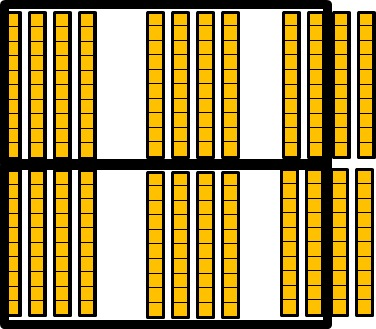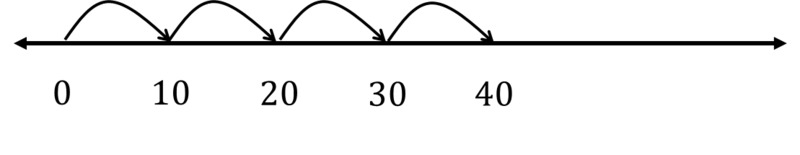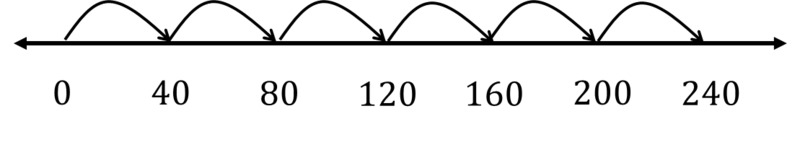# How Many Colored Pencils?

Alignments to Content Standards: 3.NBT.A.3

There are 6 tables in Mrs. Potter's art classroom. There are 4 students sitting at each table. Each student has a box of 10 colored pencils.

(A) How many colored pencils are at each table?

(B) How many colored pencils do Mrs. Potter's students have in total?

## IM Commentary

The purpose of this task is to support students' reasoning based on place value to multiply a single digit number by a multiple of 10. This reasoning builds on and supports knowledge described in 2.NBT.A.1, 3.OA.A.1, and 3.OA.B.5. If used in an instructional setting, students might benefit from having access to base-ten blocks. Students should also be encouraged to use a number line marked with tens. An empty number line is an excellent tool that helps students who are ready to develop a more abstract understanding of place value but are not yet ready for a purely symbolic solution approach.

## Solutions

Solution: 1

1. There are 4 students each with ten pencils at each table.There are 10, 20, 30, 40 pencils all together.

2. There are 6 tables with 4 boxes with ten pencils each.We can combine the 4 boxes from 2 tables to get 8 boxes, and then take 2 from one table to get 10 boxes. We can do this twice.This means there are 2 groups of 10 boxes which is 200 pencils, with 4 groups of ten pencils in addition to that.So there are 240 pencils all together.

Solution: 2

1. Each student has a box of 10 pencils, which is one group of 10. There are 4 students at each table, so there are 4 groups of 10 pencils or $4\times 10$ pencils at each table. We also know that the "4" in the number 40 means "4 tens" so we know there are 40 pencils at each table.

2. First, there are $4\times 10$ pencils at each table. Since there are 6 tables, and 40 pencils at each table, there are $6\times 40$ pencils in total. This 40 arose as 4 groups of 10, or, $4\times 10$, so there are $$6\times (4\times 10)$$ pencils in total. By the associative property of multiplication, this is also $(6\times 4)\times 10 = 24\times 10$ pencils. 24 tens is 240, so there are 240 pencils in total.

Solution: 3

1. There are 40 pencils at one table.2. There are 240 pencils all together.